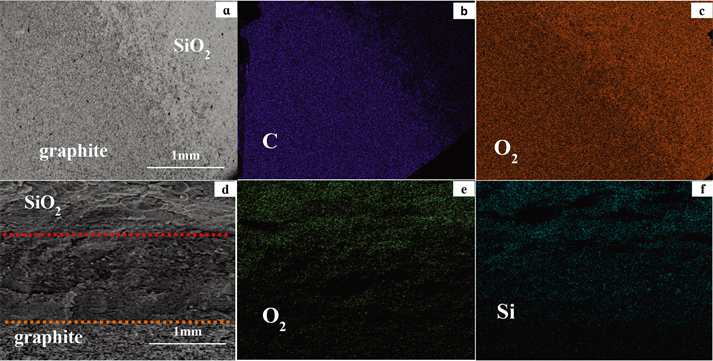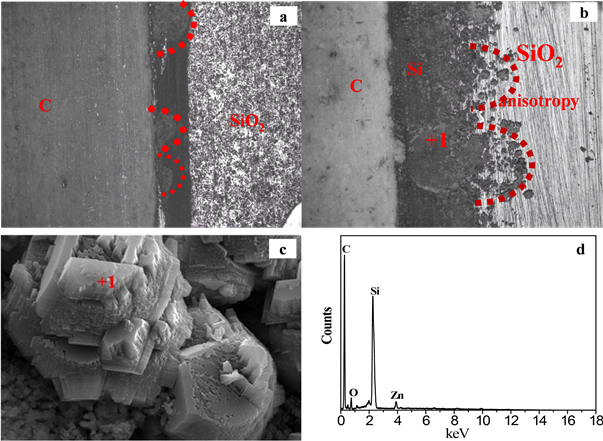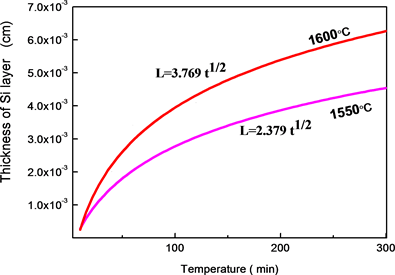﻿ 碳热还原二氧化硅过程的机理分析

碳热还原二氧化硅过程的机理分析Mechanism Analysis of Carbothermal Reduction Process of Silicon Dioxide

Abstract: In this paper, the Carbothermal reduction mechanism of SiO2 at high temperature is studied under diffusion couple of C(graphite) and SiO2. It was found that the solid reaction of SiO2 + C(graphite) = Si + CO is the main reduction process. The gasification reaction of C suppresses other reaction between SiO2 and C(graphite). The relation between Si layer thickness and time shows that the linear growth mechanism is dominant in the initial 2 h process, the slope is close to 1, and the reaction is con-trolled by the interfacial reaction; However, when the appearance thickness exceeds a critical value, the growth layer grows in a parabolic mechanism, slope is close to 0.5, and the reaction is controlled by the C diffusion.

1. 引言

2. 实验材料及方法

3. 结果与分析

3.1. SiO2与C间的反应Figure 1. Back scattered SEM image for the cross section of the SiO2(S)=C(S|graphite) diffusion couple for 3 min (a) (b) (c), 20 min (d) (e) (f) and EDS elemental mapping across the SEM image

${\text{SiO}}_{\text{2}}+\text{3C}\to \text{SiC}+\text{2CO}\Delta {\text{G}}_{\theta \text{1873}}=-\text{9}.0\text{73}$ (1)

${\text{SiO}}_{\text{2}}+\text{2C}\to \text{Si}+\text{2CO}\Delta {\text{G}}_{\theta \text{1873}}=-\text{11}.\text{3}0\text{3}$ (2)

${\text{SiO}}_{\text{2}}+\text{C}\to \text{SiO}\left(\text{g}\right)+\text{CO}\Delta {\text{G}}_{\theta \text{1873}}=-\text{9}.\text{56}$ (3)

${\text{SiO}}_{\text{2}}+\text{2SiC}\to \text{3Si}+\text{2CO}\Delta {\text{G}}_{\theta \text{1873}}=-\text{7}.\text{56}$ (4)

3.2. Si的生成与长大

SiO2(S)=C(S|石墨)扩散偶在炉内进行高温焙烧后的断面照片如图2所示。图2(a)，图2(b)内均可以观察到Figure 2. Profile structure the of SiO2(S)=C(S|graphite) diffusion couple after roasting. (a) SiO2(S)=C(S|石墨) diffusion couple 20 min, (b) SiO2(S)=C(S|石墨) diffusion couple 120 min

SiO2和C(S|石墨)未接触的地方仍保持镜面，接触处发生了SiO2和C(S|石墨)间的化学反应，对比图2(a)、图2(b)可以观察到生成层随反应时间而增厚。图2(c)为图2(b)中反应区域内+1处的高倍数SEM图片，反应产物晶体形貌明显，结合能谱数据可以认定反应生成物为Si。图2(a)为扩散偶20 min焙烧断面图，在最初的20 min的焙烧过程中，生成物Si层厚均匀，层厚变化范围不大；图2(b)为扩散偶120 min焙烧断面图，层厚变化范围较大，在SiO2一侧界面呈现出波浪状，波浪状的曲面形状是由于反应各阶段形成了不同方向的Si晶体，从而导致C(S|石墨)扩散的各向异性所引起的。图3为焙烧120 min SiO2(S)=C(S|石墨)扩散偶中SiO2试样片内不同深度中生成物Si的Debye-Scherrer图，位于远离C(石墨)一侧的Si晶体，比临近C(石墨)侧的Si晶体具有较弱的111、220、311反射。矿物内的SiO2晶体在自然形成过程中存在一定的生长方向，沿SiO2晶体生长的方向其组织致密，孔隙较少；而SiO2晶体生长方向之间空隙较多；C(S|石墨)还原SiO2晶体而得到的Si晶体因此也存在晶相差异，使的C(S|石墨)在生成物Si内的扩散就产生了方向性，扩散到反应界面上的C(S|石墨)的数量就会产生不同，会导致反应界面上生成速度的不一致，这种现象随着Si晶体厚度的增加而加剧。

$\delta ={\gamma }_{0}\cdot {\epsilon }^{\beta \cdot \left[1-\frac{1}{\left(1-{\xi }_{0}\psi \right)}\right]}$ (1)Figure 3. Debye-Scherrer XRD of Si in different deep in SiO2(S)=C(S|graphite) diffusion couple

${v}_{o}={\psi }_{mg\cdot {S}_{0}}{\chi }_{o}^{\alpha }{K}_{i}\frac{{C}_{\text{CO}}-{C}_{{\text{CO}}_{2}}/K}{\delta \frac{{k}_{i}}{{D}_{e}^{i}}+1}$ (2)Figure 4. Change of Si generation layerin SiO2(S)=C(S|graphite) diffusion coupleFigure 5. The morphology of the reactant Si and the schematic diagram of the mass transfer between SiO2 (S) and C(S| graphite)

3. 结论

1) SiO2的还原受到C的扩散过程的影响，同时C的布都尔反应会影响C还原SiO2的还原过程。

2) 弱氧化性气氛下，SiO2的还原初期由界面反应SiO2+2C→Si+2CO控制；中后期由C的扩散过程控制。

3) 生成物Si的层厚在最初的2 h线性生长机理占优势，超过临界值后，生成层以抛物线机理生长。

 何凯, 段滋华. 硅石的化学加工[J]. 中国非金属矿工业导刊, 2006(1): 47-49.

 罗旭东, 曲殿利, 张国栋, 王闯, 钟鑫宇. Al2O3对低品位菱镁矿与天然硅石合成制备镁橄榄石的影响[J]. 人工晶体学报, 2012, 41(2): 496-501.

 Anitha, C., Azim, S.S. and Mayavan, S. (2017) Fluorine Free Superhydrophobic Surface Textured Silica Particles and Its Dynamics—Transition from Impalement to Impingement. Journal of Alloys and Compounds, 3, 338-344.
https://doi.org/10.1016/j.jallcom.2017.03.338

 Shi, Z., Shi, C., Wan, S. and Ou, Z. (2017) Effect of Alkali Dosage on Alkali-Silica Reaction in Sodium Hydroxide Activated Slag Mortars. Construction and Building Materials, 3, 125-132.
https://doi.org/10.1016/j.conbuildmat.2017.03.125

 鄢捷年, 王富华. 表面活性剂吸附引起的硅石润湿性改变[J]. 油田化学, 1993(3): 195-200.

 李烨, 王志, 马文会, 孙丽媛. 硅石焙烧–酸浸提纯及杂质相结构的演变[J]. 过程工程学报, 2014, 14(3): 450- 455.

 高振昕, 张巍, 郑小平, 李君霞, 傅秋华. 山西石英岩的结晶特征与加热相变[J]. 耐火材料, 2016, 50(4): 315-320.

 马栓, 袁守谦, 唐洋洋, 王贞, 任宇君. 铁硅石代替钢屑冶炼FeSi75的试验研究[J]. 铁合金, 2014, 45(5): 17-20.

 孟德川, 王宁会, 李国锋. 黑铅硅石复合材料的电导率特性研究[J]. 功能材料, 2013, 44(6): 840-842.

 Leemann, A., Le Saout, G., Winnefeld, F., Rentsch, D. and Lothenbach. B. (2011) Alkali-Silica Reaction: The Influence of Calcium on Silica Dissolution and the Formation of reaction Products. Journal of the American Ceramic Society, 94, 1243-1249.
https://doi.org/10.1111/j.1551-2916.2010.04202.x

 何凯. 硅石加工系列报道之三——硅石制备晶体硅[J]. 中国非金属矿工业导刊, 2005(6): 49-51.

 张士轩. 去除硅石中气液包裹体的研究[J]. 渤海大学学报(自然科学版), 2002, 23(3): 13-15.

 张朝晖, 宋世雄, 巨建涛, 刘千帆. 硅石粉和焦粉粘结压块试验[J]. 钢铁研究, 2009, 37(3): 11-13.

 张慧星. 工业硅定向凝固提纯研究[D]: [硕士学位论文]. 大连: 大连理工大学, 2009.

Top